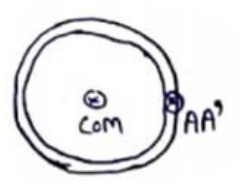# Solve the following :`
Question:

Find the radius of gyration of a circular ring of radius $r$ about a line perpendicular to the plane of the ring passing through one of its particles.

Solution:.$I_{a x i s}=I_{C O M}+m x^{2}$

$I_{A A^{r}}=m r^{2}+m r^{2}$

$I_{A A^{r}}=2 m r^{2}$

$\mathrm{~m}^{k^{2}}=2 m r^{2}$

$\therefore \mathrm{k}=\sqrt{2} r$